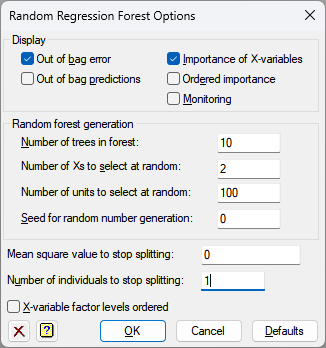1. Home
2. Random Regression Forest Options

# Random Regression Forest Options

Use this to select different options to be used in constructing the Random Regression Forest and the displayed output.## Display

Specifies which items of output are to be displayed in the Output window.

 Out of bag identifications Out of bag identifications of the groups Out of bag error Out of bag error (the square root of the mean of the squared differences of the predictions from the values in the response variate) Importance of X-variables The importance of the X-variables in the selected trees in the forest Ordered importance The importance of the X-variables displayed in decreasing order Monitoring Monitoring information during the construction process

## Random forest generation

The following four settings control how the random forest is generated.

## Number of trees in forest

Specifies the number of random trees to form in the forest. Using a larger value may improve the precision, but will take longer to generate.

## Number of Xs to select at random

Specifies the number of variables to select randomly from the X-variables list on the main menu for each tree. This must be a positive number less than the number of variables.

## Number of units to select at random

Specifies the number of units to select randomly from the observations for each tree. A usual choice would be a value corresponding to between %50 and 90% of the observations, with a typical value being 67%. This must be a positive number less than the number of observations in the variables.

## Seed for random number generation

This gives a seed to initialize the random number generation used for the random selections of variates and units. Using zero initializes this from the computer’s clock, but specifying an nonzero value gives a repeatable analysis.

## Mean square value to stop splitting

Specifies the limit on the mean square of the observations at a node at which to stop making splits.

## Number of individuals to stop splitting

Specifies the limit on the number of individuals in a group at which to stop selecting tests.

## X-variable factor levels ordered

Specifies whether the x-variable factor levels are ordered.